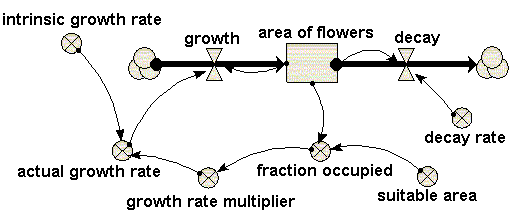# Model entry

Area covered in “flowers” - Model catalogue - Simulistics.com

 Search Simulistics Model catalogue Listed by keyword Listed by ID Listed by title Listed by date added

# Area covered in “flowers”

Model : flowers1
Simile version : 3.1+
Keywords : Area (modelling changes in) ; Vegetation dynamics ; Lumped model ;

## Description

This models the spatial spread of vegetation over an area. Here, the vegetation is taken to be “flowers”. This is not a spatial model in the usual sense where space is divided up into discrete units. Rather, the area covered by the flowers is represented by a single state variable, with an inflow representing increase in area (spread), and an outflow representing decrease in area (death of flowers).

## Diagram## Equations

```Compartments:
area of flowers
Initial value = 10
Rate of change =  + growth + decay
Comments: hectares (OK, Ford says acres, but we are in the 21st century now).

Flows:
decay = area_of_flowers*decay_rate

growth = area_of_flowers*actual_growth_rate

Variables:
actual growth rate = intrinsic_growth_rate*growth_rate_multiplier

fraction occupied = area_of_flowers/suitable_area

growth rate multiplier = graph(fraction_occupied)

Parameters:
decay rate = 0.2

intrinsic growth rate = 1

suitable area = 1000
Comments: hectares (see 'area of flowers'...)

```

## Results

|

These plots illustate changes in the flows, growth and decay, and the resulting net impact on the area of flowers.|

## References

Ford, A (1999) Modeling the Environment.
Island Press. p.58